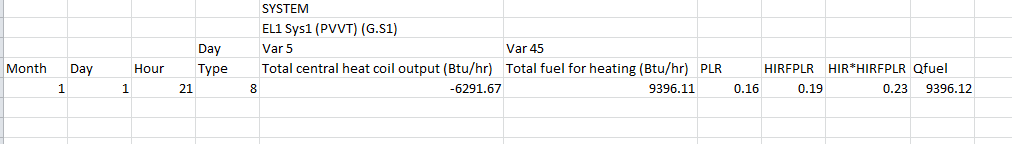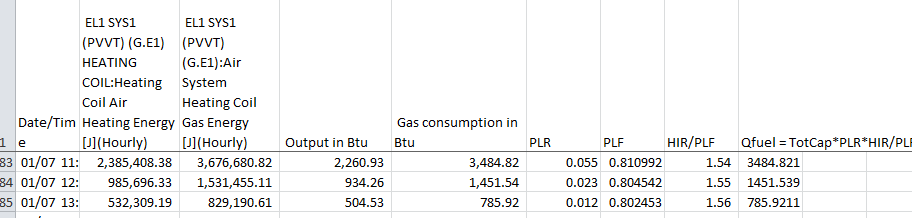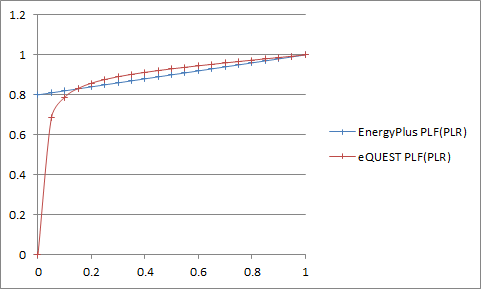Question-and-Answer Resource for the Building Energy Modeling Community
Get started with the Help page

Revision history [back]

A little update as it might be useful for other users.

Neal's answer made sense the way he presented it. However, it didn't quite add up with the data I had. To find out what the calculation is in both cases, I generated hourly reports in both eQUEST and EnergyPlus. Here is what I found out:

Calculation of the fuel consumption in DOE-2:

• Calculation of the PLR: PLR = (Load for the hour) / (Total Furnace Capacity)
• Use the PLR to get the HIR f(PLR) using the performance curve attached to the system
• Calculation of the operational HIR: HIR-op = HIR f(PLR) * HIR-rated
• Fuel consumption: Qfuel = (Total Furnace Capacity) * HIR-op (eq. 1)

Here is an excerpt from an hourly report from eQUEST that illustrate the calculation:In this example, the total furnace capacity is 39988 Btu/h and the rated HIR is 1.25.

Calculation of the fuel consumption in EnergyPlus:

• Calculation of the PLR: PLR = (Load for the hour) / (Total Furnace Capacity)
• Use the PLR to get the PLF f(PLR) using the performance curve attached to the system
• Fuel consumption: Qfuel = (Total Furnace Capacity) * PLR * HIR-rated / PLF (eq. 2)

Here is an excerpt from an hourly report from EnergyPlus that illustrate the calculation:In this example, the total furnace capacity is 39988 Btu/h and the rated HIR is 1.25.

Comparison:

Then, if we look at (eq. 1) and (eq. 2):

• HIR f(PLR) * HIR-rated = PLR * HIR-rated / PLF
• Which simplifies to HIR f(PLR) = PLR / PLF
• Thus PLF = PLR / HIR f(PLR)

In conclusion PLF and HIR f(PLR) are not quite the same.

Using this relation I converted the curve used in eQUEST (by default) to the match the PLF used in EnergyPlus.

• EnergyPlus PLF = f(PLR) is the default curve used in EnergyPlus
• eQUEST PLF = f(PLR) is the default curve used in eQUEST converted to the EnergyPlus "format".